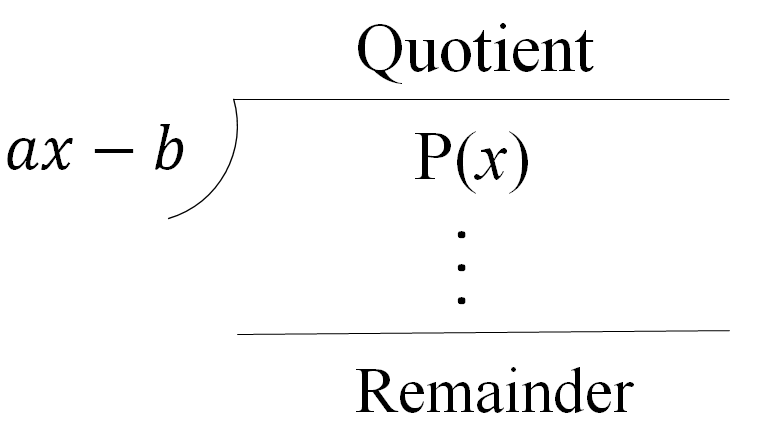# Remainder theorem#### All in One Place

Everything you need for better grades in university, high school and elementary.#### Learn with Ease

Made in Canada with help for all provincial curriculums, so you can study in confidence.#### Instant and Unlimited Help

0/4
##### Examples
###### Lessons
1. Understanding the remainder Theorem
Prove the Remainder Theorem1. Finding the Remainder Using Synthetic Division and the Remainder Theorem
Find the remainder when $\left( {4{x^3} - 7x + 10} \right)$ is divided by $\left( {2x - 5} \right)$
1. Using synthetic division
2. Using the remainder theorem
2. When $\left( {8{x^3} + a{x^2} + bx - 1} \right)$ is divided by:
i) $\left( {2x - 5} \right)$, the remainder is $54$
ii) $\left( {x + 1} \right)$, the remainder is $- 30$
Find the values of $a$ and $b$.
###### Topic Notes
You may want to refresh your memory on polynomial long division and synthetic division to better understand this lesson. The remainder theorem simply states that if a polynomial f(x) is divided by a linear expression x-r, the value of f(r) is equal to the remainder.
$\cdot$ When a polynomial, $P(x)$, is divided by $(x-a)$: Remainder $=P(a)$
$\cdot$ When a polynomial, $P(x)$, is divided by $(ax-b)$: Remainder $=P(\frac{b}{a})$